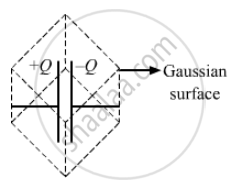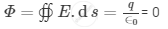Department of Pre-University Education, Karnataka course PUC Karnataka Science Class 12
Share

# A Capacitor of Capacitance C is Charged to a Potential V. the Flux of the Electric Field Through a Closed Surface Enclosing the Capacitor is - Physics

ConceptCapacitors and Capacitance

#### Question

A capacitor of capacitance C is charged to a potential V. The flux of the electric field through a closed surface enclosing the capacitor is

• (CV)/∈_0

• (2CV)/∈_0

• (CV)/(2∈_0)

• Zero

#### Solution

ZeroSince the net charge enclosed by the Gaussian surface is zero, the total flux of the electric field through the closed Gaussian surface enclosing the capacitor is zero.Here ,

Φ = Electric flux

q = Total Charge enclosed by the Gaussian surface .

Is there an error in this question or solution?

#### Video TutorialsVIEW ALL 

Solution A Capacitor of Capacitance C is Charged to a Potential V. the Flux of the Electric Field Through a Closed Surface Enclosing the Capacitor is Concept: Capacitors and Capacitance.
S